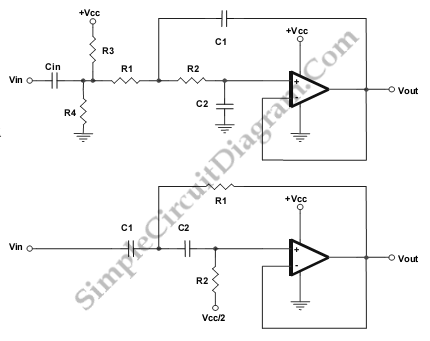# Low- and High-Pass Single Supply Sallen-Key Filter

This is a circuit of Low- and High-Pass Single Supply Sallen-Key Filter. This circuit uses popular second-order topologies, the Sallen-Key topology, because it has some advantages such as just need one a single op amp and four passive components, and it is cheap topology. Besides that, this topology is very easy to tune the frequency. However, it difficult to change filter style from Butterworth to Chebyshev.  Here is the schematic diagram of the circuit:The component values are determined by following equation:

LOW PASS

Unity Gain Butterworth

R1 = R2

R3 = R4 (HIGH)

C1 = 2C2

Fo =  squareroot(2)/ (4piR1C2)

HIGH PASS

Unity Gain Butterworth

R = 2R1

R1 = R

C1 = C2

Fo = squareroot(2) / (4piR1C1)

When we change the gain of the circuit, the style and filter tuning will  also change. Butterworth style is the easiest unity gain that we can implement a Sallen-Key filter. [Source: Texas Instruments Application Note]

The component values are determined by following equation:

LOW PASS

Unity Gain Butterworth

R1 = R2

R3 = R4 (HIGH)

C1 = 2C2

Fo =/ (4pR1C2)

HIGH PASS

Unity Gain Butterworth

R = 2R1

R1 = R

C1 = C2

Fo =/ (4pR1C1)